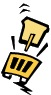# Identification of Desertification Prone Areas - Principles and General Procedures## Six steps for defining desertification prone areas

Land degradation involves a complex set of processes or factors, which interact in space and time leading to a decrease in land productivity. Thus, it is necessary to identify the various indicators, which will provide the relevant information to define the desertification prone areas.

Many aspects should be considered. However, if every possible variable has to be measured then it is unlikely that the analytical work will be completed. Therefore, as a first step the analytical work should concentrate on examining major factors affecting land degradation. Once these have been identified more detailed analysis can be undertaken. The following six procedural steps provides a guidance for using the the major factors causing desertification to define the sensitive or desertification prone areas.

## Step 1:

Identification of the indices/factors and the necessary data needed to derive the indices. Four essential considerations have to be taken into account when selecting these factors:

• The correlation to the degradation phenomena or the environmental critical state;
• Availability of data at a suitable and compatible scale ;
• Existence or ease of extrapolation from existing data sources;
• Ease and affordability to update the information, especially if it is to be used for monitor desertification process.

## Step 2:

Elaborate a model where all the indicators are listed, including the relevant data needed to calculate them. Define the relationships that connect all the data and indicators.

## Step 3:

Map all the initial data into data layers, which could be overlaid in a GIS (Geographical Information System).

## Step 4:

Apply a re-classification scheme with values ranging from 1-2 to each component of the initial data layers. The value "1" is assigned to components which contribute least to desertification while "2" is assigned to components which contribute most. The range between 1 and 2 reflects relative role.

## Step 5:

Calculate each index based on the pertinent data layers. Each index-layer is the result of the overlay process in the GIS of the different relevant data layers and it is estimated as the geometric mean of its own sub-layers, according to the following formula:

Index_x = (layer_1 x * layer_2 x * layer_3 x * ...... * layer_n x) (1/n)

Where x is the index in consideration and n is the number of layers used for a specific index.

## Step 6:

Calculate the DPA (Desertification Prone Areas). The DPA is the result of the overlay process in GIS of the different indices and is estimated by the geometric mean of the individual indices:

DPA = (index_1 * index_2 * index_3 * ...... * index_n) (1/n)

Where n is the number of indices used for calculation of DPA.Figure 1: The DPA model approach